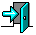# CS3013 Practice Mid-Semester Exam

1. Explain why a Micro-Kernel architecture is one of the easiest OS structures to extend to a distributed system.

2. What are the various fields that need to be saved in a process control block (PCB)? Do we have to save the general purpose registers? Why or why not?

3. In class, we noted that the `Dispatcher()` function does not return in the normal fashion. What did we mean by that?

4. What are the possible outputs when the following code is compiled and run simultaneously in two separate processes on a Unix system (note that the code below is not identical to typical Unix system calls)?

```    #include < stdio.h >
#include "shm.h"		/* for our shared mem/sem primatives */

int main(int argc, char *argv[]) {
int *num;

num = (int *) shmattach(502); /* attach to shared memory */
if (num == NULL) {  /* if attach fails */
num = (int *) shmcreate(502, sizeof(int));  /* create shared memory */
*num = 0; /* initialize to 0 */
}
*num = *num - 1;
printf("%d\n", *num);

}
```

5. Five processes are ready to run. Their CPU burst times are 5, 12, 1, 7 and 2. In what order are they run under a FCFS scheduling algorithm? What is the average waiting time? How close is this to the best average waiting time we could have had for these processes? What is the throughput?

6. Modify Peterson's Solution as shown in class to properly synchronize 3 processes instead of only 2. You can add shared variables as needed.

7. True or False:

1. Conditional critical regions allow you to solve more synchronization problems than do semaphores.
2. You will never get deadlock if you use semaphores.
3. A process may still be starved in a correct critical region solution.
4. Disabling interrupts, even by the operating system, is always a bad idea.
5. Monitors are more commonly provided by today's operating systems than are semaphores.

8. In the dining philosopher's solution shown in class, suppose philosopher 2 was absentminded and never let go of his left fork, even while thinking. If all the other philosophers behaved normally and kept thinking and eating, what would be the eventual outcome of the system?

9. Modify the dining philosopher's solution shown in class to have the "odd" philosophers pick up their "left" forks first and the "even" philosophers pick up their "right" forks first. Does this solve the problem of potential deadlock?

10. Threads are often called "lightweight" processes. Why? What is "light" about them?

11. Consider the `StartUsingProcessTable()` function for the Simple Operating System that we looked at in class. Is there busy waiting? If so, why is it ok here? If not, what kind of waiting is it?

12. Consider the following incomplete solution for a basic Unix shell:

```      while (1) {

     _A____

if (id>0) {

       _______

       _______

} else {

       _______

       _______

       _______
}
}

```

Indicate where each of the following pseudo-system calls should go in the above code. The first one has been done for you:

```  A. id = fork()
B. call execvp(cmd, args)
C. wait()
D. print out "this is the child process"
E. print out "this is the parent process"
F. exit()
```

13. Given the template for a `WaitSemaphore()` system call:

```/* Do a semaphore wait on the semaphore indicated by sid */
int WaitSemaphore(int sid) {
/* statements a-h here */
}
```

Put the following statements in the right order:

``` return 0; endif if (sema[sid].count < 0) then sema[sid].count--; pd[current_process].state = BLOCKED; if (!sema[sid].allocated) return -1; Dispatcher(); ```
1. ```insert(sema[sid].queue, current_process); ```Return to the CS3013 Home Page